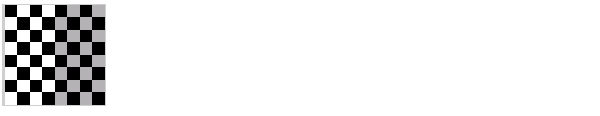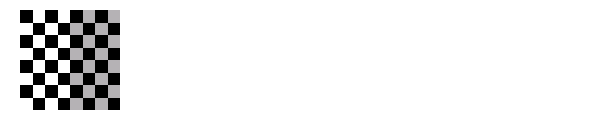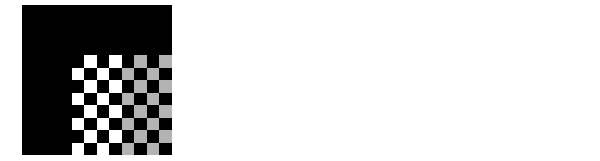Image Processing Toolbox User's GuideExample: Performing a Translation

This example illustrates how to use the `maketform` and `imtransform` functions to perform a 2-D spatial transformation of an image. The example performs a simple affine transformation called a translation. In a translation, you shift an image in coordinate space by adding a specified value to the x- and y-coordinates. The example illustrates the following steps:

Step 1: Import the Image to Be Transformed

Bring the image to be transformed into the MATLAB workspace. This example creates a checkerboard image, using the `checkerboard` function. By default, checkerboard creates an 80-by-80 pixel image.

• ```cb = checkerboard;
imshow(cb)```

Original Image

Step 2: Define the Spatial Transformation

You must define the spatial transformation that you want to perform. For many types of 2-D spatial transformations, such as affine transformations, you can use a 3-by-3 transformation matrix to specify the transformation. You can also use sets of points in the input and output images to specify the transformation and let `maketform` create the transformation matrix. For more information, see Defining the Transformation Data.

This example uses the following transformation matrix to define a spatial transformation called a translation.

• ```xform = [ 1  0  0
0  1  0
40 40  1 ]
```

In this matrix, `xform(3,1)` specifies the number of pixels to shift the image in the horizontal direction and `xform(3,2)` specifies the number of pixels to shift the image in the vertical direction.

Step 3: Create the TFORM Structure

You use the `maketform` function to create a `TFORM` structure. As arguments, you specify the type of transformation you want to perform and the transformation matrix (or set of points) that you created to define the transformation. For more information, see Creating TFORM Structures.

This example calls `maketform`, specifying `'affine'` as the type of transformation, because translation is a type of affine transformation, and `xform`, the transformation matrix created in step 2.

• ```tform_translate = maketform('affine',xform);
```

Step 4: Perform the Transformation

To perform the transformation, call the `imtransform` function, specifying the image you want to transform and the `TFORM` structure that stores all the required transformation parameters. For more information, see Performing the Spatial Transformation.

The following example passes to the `imtransform` function the checkerboard image, created in Step 1, and the `TFORM` structure created in Step 3. `imtransform` returns the transformed image.

• ```[cb_trans xdata ydata]= imtransform(cb, tform_translate);
```

The example includes two optional output arguments: `xdata` and `ydata`. These arguments return the location of the output image in output coordinate space. `xdata` contains the x-coordinates of the pixels at the corners of the output image. `ydata` contains the y-coordinates of these same pixels.

 Note    This section uses the spatial coordinate system when referring to pixel locations. In the spatial coordinates system, the x- and y-coordinates specify the center of the pixel. For more information about the distinction between spatial coordinates and pixel coordinates, see Coordinate Systems.

The following figure illustrates this translation graphically. By convention, the axes in input space are labeled u and v and the axes in output space are labelled x and y. In the figure, note how `imtransform` modifies the spatial coordinates that define the locations of pixels in the input image. The pixel at (1,1) is now positioned at (41,41). (In the checkerboard image, each black, white, and gray square is 10 pixels high and 10 pixels wide.)Input Image Translated

Pixel Values and Pixel Locations.   The previous figure shows how `imtransform` changes the locations of pixels between input space and output space. The pixel located at (1,1) in the input image is now located at (41,41) in the output image. Note, however, that the value at that pixel location has not changed. Pixel (1,1) in the input image is black and so is pixel (41,41) in the output image.

`imtransform` determines the value of pixels in the output image by mapping the new locations back to the corresponding locations in the input image (inverse mapping). In a translation, because the size and orientation of the output image is the same as the input image, this is a one to one mapping of pixel values to new locations. For other types of transformations, such as scaling or rotation, `imtransform` interpolates within the input image to compute the output pixel value. For more information about the interpolation methods used by `imtransform`, see Interpolation.

Step 5: View the Output Image

After performing the transformation, you might want to view the transformed image. The example uses the `imshow` function to display the transformed image.

• ```figure, imshow(cb_trans)```

Translated Image

Understanding the Display of the Transformed Image.   When viewing the transformed image, especially for a translation operation, it might appear that the transformation had no effect. The transformed image looks identical to the original image. However, if you check the `xdata` and `ydata` values returned by `imtransform`, you can see that the spatial coordinates have changed. The upper left corner of the input image with spatial coordinates (1,1) is now (41,41). The lower right corner of the input image with spatial coordinates (80,80) is now (120,120). The value 40 has been added to each, as expected.

• ```xdata =

41   120

ydata =

41   120
```

The reason that no change is apparent in the visualization is because `imtransform` sizes the output image to be just large enough to contain the entire transformed image but not the entire output coordinate space. To see the effect of the translation in relation to the original image, you can use several optional input parameters that specify the size of output image and how much of the output space is included in the output image.

The example uses two of these optional input parameters, `XData` and `YData`, to specify how much of the output coordinate space to include in the output image. The example sets the `XData` and `YData` to include the origin of the original image and be large enough to contain the entire translated image.

 Note    Note how all the pixels that are now in the output image that do not correspond to locations in the input image are black. `imtransform` assigns a value, called a fill value, to these pixels. This example uses the default fill value but you can specify a different one -- see Specifying Fill Values

• ```cb_trans2 = imtransform(cb, tform_translate,...
'XData',[1 (size(cb,2)+xform(3,1)],...
'YData', [1 (size(cb,1)+xform(3,2)]);
figure, imshow(cb_trans2)```

View of the Translated Image in Relation to Original Coordinate SpacePerforming General 2-D Spatial Transformations Defining the Transformation Data